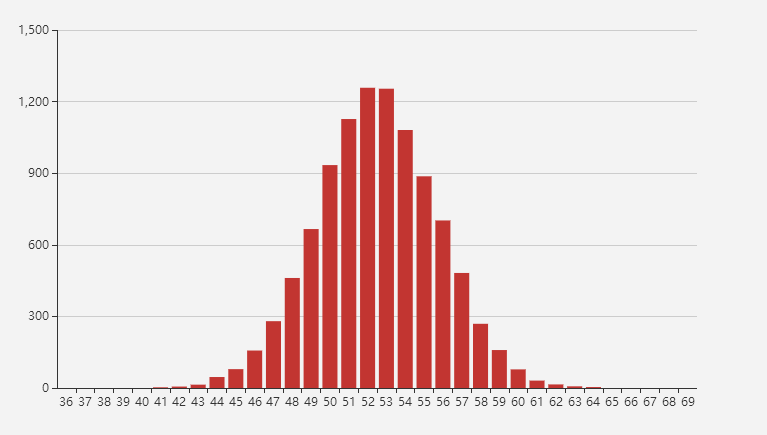# java产生服从正态分布的随机数

C.F.高斯在研究测量误差时从另一个角度导出了它。P.S.拉普拉斯和高斯研究了它的性质。

JDK中Random类中的nextGaussian()方法，可以产生服从标准正态分布的随机数。即 X~N(0,1);

``````Math.sqrt(b)*random.nextGaussian() + μ;
``````

``````Random r = new Random();
for (int i = 0; i < 10000; i++) {
r.nextGaussian() * Math.sqrt(10) + 53;
}
``````微信打赏